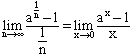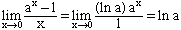Name : Yannick Gigandet How can I solve these two limits : lim when n approches 1 of n[a1/n -1] lim when x approches 0 of (eax - ebx) / x Thanks for the answer! Hi Yannick, I think in the first problem you want the limit as n approaches infinity. In both cases the way to find these limits is to use l'Hospital's Rule. For the first example write n[a1/n -1] as [a1/n -1]/[1/n]. I wouls then make the change of variables, x = 1/n to getNow apply l'Hospital's Rule by differentiating both the numerator and denominator to getCheers Harley Go to Math Central